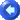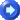AssetsAssets

Assets are the definitions or profiles of the materials, equipment, etc. you are tracking. Assets can be broadly divided into user-defined types, such as equipment, materials or expenses (see the Asset Types topic for more information). Assets can be further divided into user-defined classes, for example equipment assets may be classified by Truck, Auto, etc. (see the Asset Classes topic for more information).

Assets define what you keep track of, whereas Asset Entries are used to track the actual usage of these assets. For example, you may have a material asset named 'Gravel', and an asset entry that indicates how much gravel a crew used on a particular date. See the Asset Entries topic for more information.

 Note: You can change the terminology 'Asset' to suit your organization's needs. The Terminology topic has more information.

Assets have the following properties:

Property

Description

Name

The asset name, up to 80 characters. A value is required and must be unique for all assets (regardless of the asset type).

Description

A description for the asset, up to 255 characters.

ID

The asset ID, up to 80 characters. This value is not required, but if a value is entered it must be unique for all assets (regardless of the asset type). This is commonly used for synchronizing with external systems, such as billing or accounting.

Status

The asset status, either Active or Inactive. Setting the status to inactive will prevent the recording of any further asset entries for this asset. Note that you cannot delete assets that have asset entries associated with them, and in those cases you will instead make the asset inactive to prevent its further use.

Class

The asset class (optional). Asset classes are configured on the Assets > Asset Class page (see the Asset Classes topic form more information).

Unit of Measure

The unit of measure for this asset (optional). Units of measure are configured on the Assets > Units of Measure page (see the Units of Measure topic form more information).

Units Decimal Digits

The number of decimal digits of precision for entering the quantity value for asset entries. It will default to the "Default Decimal Digits" property for the selected unit of measure, but you can override this.

Unit Amount

The unit amount or cost of this asset, per unit of measure. Amount is what you pay for this asset, as opposed to the bill amount, which is what you charge clients or customers for this asset. Unit Amount is used to compute the total amount based on the quantity entered by the employee. The unit amount is only useful if you have the employee enter the quantity.

If multi-currency is enabled for the asset type then you can also select the default currency for this asset.

Unit amounts can be editable by employees, or fixed (for example, you may have a Mileage item that has a fixed rate of 0.55 per mile and you do not want the employee to change that rate).

You can also control whether the unit amount is editable (as well as total amount) by setting the entry field permissions (see the Asset Entry Fields topic for more information).

Unit Bill Amount

The unit bill amount or rate for this asset, per unit of measure. Bill amount is what you charge for this asset, as opposed to cost, which is what you pay for this asset. Unit bill amount is used to compute the total bill amount based on the quantity entered by the employee. The unit bill amount is only useful if you have the employee enter the quantity.

Unit bill amount can be either a fixed value, or can be computed. The following options are available:

 Fixed Amount The unit price is always the value entered: it does not change based on the unit cost. Markup The unit bill amount is computed from the unit cost as a percent markup: Unit Bill Amount = Unit Cost * (1 + Markup) For example, if the markup is 50% and the unit cost is \$10.00, then the unit bill amount will be \$15.00. Margin The unit bill amount is computed from the unit cost to give you a percent margin: Unit Bill Amount = Unit Cost / (1 - Margin) For example, if the margin is 50% and the unit cost is \$10.00, then the unit bill amount will be \$20.00. As you can see from the equation, the margin can never be 100% or greater.

Note that you can optionally allow entries to override this unit bill amount if you allow the entry's Unit Bill Amount or Total Bill Amount fields to be editable (see the Asset Entry Fields topic for more information).

Notes